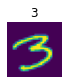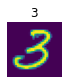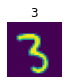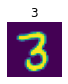# Data transformations

Functions for getting, splitting, and labeling data, as well as generic transforms

## Get, split, and label

For most data source creation we need functions to get a list of items, split them in to train/valid sets, and label them. fastai provides functions to make each of these steps easy (especially when combined with `fastai.data.blocks`).

### Get

First we’ll look at functions that get a list of items (generally file names).

We’ll use tiny MNIST (a subset of MNIST with just two classes, `7`s and `3`s) for our examples/tests throughout this page.

``````path = untar_data(URLs.MNIST_TINY)
(path/'train').ls()``````
``(#2) [Path('/Users/jhoward/.fastai/data/mnist_tiny/train/7'),Path('/Users/jhoward/.fastai/data/mnist_tiny/train/3')]``

source

### get_files

`````` get_files (path, extensions=None, recurse=True, folders=None,

Get all the files in `path` with optional `extensions`, optionally with `recurse`, only in `folders`, if specified.

This is the most general way to grab a bunch of file names from disk. If you pass `extensions` (including the `.`) then returned file names are filtered by that list. Only those files directly in `path` are included, unless you pass `recurse`, in which case all child folders are also searched recursively. `folders` is an optional list of directories to limit the search to.

``````t3 = get_files(path/'train'/'3', extensions='.png', recurse=False)
t7 = get_files(path/'train'/'7', extensions='.png', recurse=False)
t  = get_files(path/'train', extensions='.png', recurse=True)
test_eq(len(t), len(t3)+len(t7))
test_eq(len(get_files(path/'train'/'3', extensions='.jpg', recurse=False)),0)
test_eq(len(t), len(get_files(path, extensions='.png', recurse=True, folders='train')))
t``````
``(#709) [Path('/Users/jhoward/.fastai/data/mnist_tiny/train/7/9243.png'),Path('/Users/jhoward/.fastai/data/mnist_tiny/train/7/9519.png'),Path('/Users/jhoward/.fastai/data/mnist_tiny/train/7/7534.png'),Path('/Users/jhoward/.fastai/data/mnist_tiny/train/7/9082.png'),Path('/Users/jhoward/.fastai/data/mnist_tiny/train/7/8377.png'),Path('/Users/jhoward/.fastai/data/mnist_tiny/train/7/994.png'),Path('/Users/jhoward/.fastai/data/mnist_tiny/train/7/8559.png'),Path('/Users/jhoward/.fastai/data/mnist_tiny/train/7/8217.png'),Path('/Users/jhoward/.fastai/data/mnist_tiny/train/7/8571.png'),Path('/Users/jhoward/.fastai/data/mnist_tiny/train/7/8954.png')...]``

It’s often useful to be able to create functions with customized behavior. `fastai.data` generally uses functions named as CamelCase verbs ending in `er` to create these functions. `FileGetter` is a simple example of such a function creator.

source

### FileGetter

`` FileGetter (suf='', extensions=None, recurse=True, folders=None)``

Create `get_files` partial function that searches path suffix `suf`, only in `folders`, if specified, and passes along args

``````fpng = FileGetter(extensions='.png', recurse=False)
test_eq(len(t7), len(fpng(path/'train'/'7')))
test_eq(len(t), len(fpng(path/'train', recurse=True)))
fpng_r = FileGetter(extensions='.png', recurse=True)
test_eq(len(t), len(fpng_r(path/'train')))``````

source

### get_image_files

`` get_image_files (path, recurse=True, folders=None)``

Get image files in `path` recursively, only in `folders`, if specified.

This is simply `get_files` called with a list of standard image extensions.

``test_eq(len(t), len(get_image_files(path, recurse=True, folders='train')))``

source

### ImageGetter

`` ImageGetter (suf='', recurse=True, folders=None)``

Create `get_image_files` partial that searches suffix `suf` and passes along `kwargs`, only in `folders`, if specified

Same as `FileGetter`, but for image extensions.

``````test_eq(len(get_files(path/'train', extensions='.png', recurse=True, folders='3')),
len(ImageGetter(   'train',                    recurse=True, folders='3')(path)))``````

source

### get_text_files

`` get_text_files (path, recurse=True, folders=None)``

Get text files in `path` recursively, only in `folders`, if specified.

source

### ItemGetter

`` ItemGetter (i)``

Creates a proper transform that applies `itemgetter(i)` (even on a tuple)

``````test_eq(ItemGetter(1)((1,2,3)),  2)
test_eq(ItemGetter(1)(L(1,2,3)), 2)
test_eq(ItemGetter(1)([1,2,3]),  2)
test_eq(ItemGetter(1)(np.array([1,2,3])),  2)``````

source

### AttrGetter

`` AttrGetter (nm, default=None)``

Creates a proper transform that applies `attrgetter(nm)` (even on a tuple)

``````test_eq(AttrGetter('shape')(torch.randn([4,5])), [4,5])
test_eq(AttrGetter('shape', )([4,5]), )``````

### Split

The next set of functions are used to split data into training and validation sets. The functions return two lists - a list of indices or masks for each of training and validation sets.

source

### RandomSplitter

`` RandomSplitter (valid_pct=0.2, seed=None)``

Create function that splits `items` between train/val with `valid_pct` randomly.

``````def _test_splitter(f, items=None):
"A basic set of condition a splitter must pass"
items = ifnone(items, range_of(30))
trn,val = f(items)
assert 0<len(trn)<len(items)
assert all(o not in val for o in trn)
test_eq(len(trn), len(items)-len(val))
# test random seed consistency
test_eq(f(items), trn)
return trn, val``````
``_test_splitter(RandomSplitter(seed=42))``
``((#24) [10,18,16,23,28,26,20,7,21,22...], (#6) [12,0,6,25,8,15])``

Use scikit-learn train_test_split. This allow to split items in a stratified fashion (uniformely according to the ’labels‘ distribution)

source

### TrainTestSplitter

`````` TrainTestSplitter (test_size=0.2, random_state=None, stratify=None,
train_size=None, shuffle=True)``````

Split `items` into random train and test subsets using sklearn train_test_split utility.

``````src = list(range(30))
labels =  * 20 +  * 10
test_size = 0.2

f = TrainTestSplitter(test_size=test_size, random_state=42, stratify=labels)
trn,val = _test_splitter(f, items=src)

# test labels distribution consistency
# there should be test_size % of zeroes and ones respectively in the validation set
test_eq(len([t for t in val if t < 20]) / 20, test_size)
test_eq(len([t for t in val if t > 20]) / 10, test_size)``````

source

### IndexSplitter

`` IndexSplitter (valid_idx)``

Split `items` so that `val_idx` are in the validation set and the others in the training set

``````items = 'a,b,c,d,e,f,g,h,i,j'.split(',')  #to make obvious that splits indexes and not items.
splitter = IndexSplitter([3,7,9])

_test_splitter(splitter, items)
test_eq(splitter(items),[[0,1,2,4,5,6,8],[3,7,9]])``````

source

### EndSplitter

`` EndSplitter (valid_pct=0.2, valid_last=True)``

Create function that splits `items` between train/val with `valid_pct` at the end if `valid_last` else at the start. Useful for ordered data.

``````items = range_of(10)

splitter_last = EndSplitter(valid_last=True)
_test_splitter(splitter_last)
test_eq(splitter_last(items), ([0,1,2,3,4,5,6,7], [8,9]))

splitter_start = EndSplitter(valid_last=False)
_test_splitter(splitter_start)
test_eq(splitter_start(items), ([2,3,4,5,6,7,8,9], [0,1]))``````

source

### GrandparentSplitter

`` GrandparentSplitter (train_name='train', valid_name='valid')``

Split `items` from the grand parent folder names (`train_name` and `valid_name`).

``````fnames = [path/'train/3/9932.png', path/'valid/7/7189.png',
path/'valid/7/7320.png', path/'train/7/9833.png',
path/'train/3/7666.png', path/'valid/3/925.png',
path/'train/7/724.png', path/'valid/3/93055.png']
splitter = GrandparentSplitter()``````
``````_test_splitter(splitter, items=fnames)
test_eq(splitter(fnames),[[0,3,4,6],[1,2,5,7]])``````
``````fnames2 = fnames + [path/'test/3/4256.png', path/'test/7/2345.png', path/'valid/7/6467.png']
splitter = GrandparentSplitter(train_name=('train', 'valid'), valid_name='test')
_test_splitter(splitter, items=fnames2)
test_eq(splitter(fnames2),[[0,3,4,6,1,2,5,7,10],[8,9]])``````

source

### FuncSplitter

`` FuncSplitter (func)``

Split `items` by result of `func` (`True` for validation, `False` for training set).

``````splitter = FuncSplitter(lambda o: Path(o).parent.parent.name == 'valid')
_test_splitter(splitter, fnames)
test_eq(splitter(fnames),[[0,3,4,6],[1,2,5,7]])``````

source

`` MaskSplitter (mask)``

Split `items` depending on the value of `mask`.

``````items = list(range(6))
_test_splitter(splitter, items)
test_eq(splitter(items),[[1,2,4],[0,3,5]])``````

source

### FileSplitter

`` FileSplitter (fname)``

Split `items` by providing file `fname` (contains names of valid items separated by newline).

``````with tempfile.TemporaryDirectory() as d:
fname = Path(d)/'valid.txt'
fname.write_text('\n'.join([Path(fnames[i]).name for i in [1,3,4]]))
splitter = FileSplitter(fname)
_test_splitter(splitter, fnames)
test_eq(splitter(fnames),[[0,2,5,6,7],[1,3,4]])``````

source

### ColSplitter

`` ColSplitter (col='is_valid', on=None)``

Split `items` (supposed to be a dataframe) by value in `col`

``````df = pd.DataFrame({'a': [0,1,2,3,4], 'b': [True,False,True,True,False]})
splits = ColSplitter('b')(df)
test_eq(splits, [[1,4], [0,2,3]])
# Works with strings or index
splits = ColSplitter(1)(df)
test_eq(splits, [[1,4], [0,2,3]])
# does not get confused if the type of 'is_valid' is integer, but it meant to be a yes/no
df = pd.DataFrame({'a': [0,1,2,3,4], 'is_valid': [1,0,1,1,0]})
splits_by_int = ColSplitter('is_valid')(df)
test_eq(splits_by_int, [[1,4], [0,2,3]])
# optionally pass a specific value to split on
df = pd.DataFrame({'a': [0,1,2,3,4,5], 'b': [1,2,3,1,2,3]})
splits_on_val = ColSplitter('b', 3)(df)
test_eq(splits_on_val, [[0,1,3,4], [2,5]])
# or multiple values
splits_on_val = ColSplitter('b', [2,3])(df)
test_eq(splits_on_val, [[0,3], [1,2,4,5]])``````

source

### RandomSubsetSplitter

`` RandomSubsetSplitter (train_sz, valid_sz, seed=None)``

Take randoms subsets of `splits` with `train_sz` and `valid_sz`

``````items = list(range(100))
valid_idx = list(np.arange(70,100))
splitter = RandomSubsetSplitter(0.3, 0.1)
splits = RandomSubsetSplitter(0.3, 0.1)(items)
test_eq(len(splits), 30)
test_eq(len(splits), 10)``````

### Label

The final set of functions is used to label a single item of data.

source

### parent_label

`` parent_label (o)``

Label `item` with the parent folder name.

Note that `parent_label` doesn’t have anything customize, so it doesn’t return a function - you can just use it directly.

``````test_eq(parent_label(fnames), '3')
test_eq(parent_label("fastai_dev/dev/data/mnist_tiny/train/3/9932.png"), '3')
[parent_label(o) for o in fnames]``````
``['3', '7', '7', '7', '3', '3', '7', '3']``

source

### RegexLabeller

`` RegexLabeller (pat, match=False)``

Label `item` with regex `pat`.

`RegexLabeller` is a very flexible function since it handles any regex search of the stringified item. Pass `match=True` to use `re.match` (i.e. check only start of string), or `re.search` otherwise (default).

For instance, here’s an example the replicates the previous `parent_label` results.

``````f = RegexLabeller(fr'{posixpath.sep}(\d){posixpath.sep}')
test_eq(f(fnames), '3')
[f(o) for o in fnames]``````
``['3', '7', '7', '7', '3', '3', '7', '3']``
``````f = RegexLabeller(fr'{posixpath.sep}(\d){posixpath.sep}')
a1 = Path(fnames).as_posix()
test_eq(f(a1), '3')
[f(o) for o in fnames]``````
``['3', '7', '7', '7', '3', '3', '7', '3']``
``````f = RegexLabeller(r'(\d*)', match=True)
test_eq(f(fnames.name), '9932')``````

source

`` ColReader (cols, pref='', suff='', label_delim=None)``

Read `cols` in `row` with potential `pref` and `suff`

`cols` can be a list of column names or a list of indices (or a mix of both). If `label_delim` is passed, the result is split using it.

``````df = pd.DataFrame({'a': 'a b c d'.split(), 'b': ['1 2', '0', '', '1 2 3']})
test_eq([f(o) for o in df.itertuples()], '0a1 0b1 0c1 0d1'.split())

test_eq([f(o) for o in df.itertuples()], [['1', '2'], ['0'], [], ['1', '2', '3']])

df['a1'] = df['a']
f = ColReader(['a', 'a1'], pref='0', suff='1')
test_eq([f(o) for o in df.itertuples()], [L('0a1', '0a1'), L('0b1', '0b1'), L('0c1', '0c1'), L('0d1', '0d1')])

df = pd.DataFrame({'a': [L(0,1), L(2,3,4), L(5,6,7)]})
test_eq([f(o) for o in df.itertuples()], [L(0,1), L(2,3,4), L(5,6,7)])

df['name'] = df['a']
test_eq([f(df.iloc[0,:])], [L(0,1)])

test_eq([f(o) for o in df.itertuples()], [L(0,1), L(2,3,4), L(5,6,7)])
test_eq([f(df.iloc[0,:])], [L(0,1)])``````

source

### CategoryMap

`` CategoryMap (col, sort=True, add_na=False, strict=False)``

Collection of categories with the reverse mapping in `o2i`

``````t = CategoryMap([4,2,3,4])
test_eq(t, [2,3,4])
test_eq(t.o2i, {2:0,3:1,4:2})
test_eq(t.map_objs([2,3]), [0,1])
test_eq(t.map_ids([0,1]), [2,3])
test_fail(lambda: t.o2i['unseen label'])``````
``````t = CategoryMap([4,2,3,4], add_na=True)
test_eq(t, ['#na#',2,3,4])
test_eq(t.o2i, {'#na#':0,2:1,3:2,4:3})``````
``````t = CategoryMap(pd.Series([4,2,3,4]), sort=False)
test_eq(t, [4,2,3])
test_eq(t.o2i, {4:0,2:1,3:2})``````
``````col = pd.Series(pd.Categorical(['M','H','L','M'], categories=['H','M','L'], ordered=True))
t = CategoryMap(col)
test_eq(t, ['H','M','L'])
test_eq(t.o2i, {'H':0,'M':1,'L':2})``````
``````col = pd.Series(pd.Categorical(['M','H','M'], categories=['H','M','L'], ordered=True))
t = CategoryMap(col, strict=True)
test_eq(t, ['H','M'])
test_eq(t.o2i, {'H':0,'M':1})``````

source

### Categorize

`` Categorize (vocab=None, sort=True, add_na=False)``

Reversible transform of category string to `vocab` id

source

### Category

str(object=’’) -> str str(bytes_or_buffer[, encoding[, errors]]) -> str

Create a new string object from the given object. If encoding or errors is specified, then the object must expose a data buffer that will be decoded using the given encoding and error handler. Otherwise, returns the result of object.__str__() (if defined) or repr(object). encoding defaults to sys.getdefaultencoding(). errors defaults to ‘strict’.

``````cat = Categorize()
tds = Datasets(['cat', 'dog', 'cat'], tfms=[cat])
test_eq(cat.vocab, ['cat', 'dog'])
test_eq(cat('cat'), 0)
test_eq(cat.decode(1), 'dog')
test_stdout(lambda: show_at(tds,2), 'cat')
test_fail(lambda: cat('bird'))``````
``````cat = Categorize(add_na=True)
tds = Datasets(['cat', 'dog', 'cat'], tfms=[cat])
test_eq(cat.vocab, ['#na#', 'cat', 'dog'])
test_eq(cat('cat'), 1)
test_eq(cat.decode(2), 'dog')
test_stdout(lambda: show_at(tds,2), 'cat')``````
``````cat = Categorize(vocab=['dog', 'cat'], sort=False, add_na=True)
tds = Datasets(['cat', 'dog', 'cat'], tfms=[cat])
test_eq(cat.vocab, ['#na#', 'dog', 'cat'])
test_eq(cat('dog'), 1)
test_eq(cat.decode(2), 'cat')
test_stdout(lambda: show_at(tds,2), 'cat')``````

source

### MultiCategorize

`` MultiCategorize (vocab=None, add_na=False)``

Reversible transform of multi-category strings to `vocab` id

source

### MultiCategory

`` MultiCategory (items=None, *rest, use_list=False, match=None)``

Behaves like a list of `items` but can also index with list of indices or masks

``````cat = MultiCategorize()
tds = Datasets([['b', 'c'], ['a'], ['a', 'c'], []], tfms=[cat])
test_eq(tds, TensorMultiCategory([]))
test_eq(cat.vocab, ['a', 'b', 'c'])
test_eq(cat(['a', 'c']), tensor([0,2]))
test_eq(cat([]), tensor([]))
test_eq(cat.decode(), ['b'])
test_eq(cat.decode([0,2]), ['a', 'c'])
test_stdout(lambda: show_at(tds,2), 'a;c')

# if vocab supplied, ensure it maintains its order (i.e., it doesn't sort)
cat = MultiCategorize(vocab=['z', 'y', 'x'])
test_eq(cat.vocab, ['z','y','x'])

test_fail(lambda: cat('bird'))``````

source

### OneHotEncode

`` OneHotEncode (c=None)``

One-hot encodes targets

Works in conjunction with `MultiCategorize` or on its own if you have one-hot encoded targets (pass a `vocab` for decoding and `do_encode=False` in this case)

``````_tfm = OneHotEncode(c=3)
test_eq(_tfm([0,2]), tensor([1.,0,1]))
test_eq(_tfm.decode(tensor([0,1,1])), [1,2])``````
``````tds = Datasets([['b', 'c'], ['a'], ['a', 'c'], []], [[MultiCategorize(), OneHotEncode()]])
test_eq(tds, [tensor([1.,0,0])])
test_eq(tds, [tensor([0.,0,0])])
test_eq(tds.decode([tensor([False, True, True])]), [['b','c']])
test_eq(type(tds), TensorMultiCategory)
test_stdout(lambda: show_at(tds,2), 'a;c')``````

source

### EncodedMultiCategorize

`` EncodedMultiCategorize (vocab)``

Transform of one-hot encoded multi-category that decodes with `vocab`

``````_tfm = EncodedMultiCategorize(vocab=['a', 'b', 'c'])
test_eq(_tfm([1,0,1]), tensor([1., 0., 1.]))
test_eq(type(_tfm([1,0,1])), TensorMultiCategory)
test_eq(_tfm.decode(tensor([False, True, True])), ['b','c'])

_tfm2 = EncodedMultiCategorize(vocab=['c', 'b', 'a'])
test_eq(_tfm2.vocab, ['c', 'b', 'a'])``````

source

### RegressionSetup

`` RegressionSetup (c=None)``

Transform that floatifies targets

``````_tfm = RegressionSetup()
dsets = Datasets([0, 1, 2], RegressionSetup)
test_eq(dsets.c, 1)
test_eq_type(dsets, (tensor(0.),))

dsets = Datasets([[0, 1, 2], [3,4,5]], RegressionSetup)
test_eq(dsets.c, 3)
test_eq_type(dsets, (tensor([0.,1.,2.]),))``````

source

### get_c

`` get_c (dls)``

## End-to-end dataset example with MNIST

Let’s show how to use those functions to grab the mnist dataset in a `Datasets`. First we grab all the images.

``````path = untar_data(URLs.MNIST_TINY)
items = get_image_files(path)``````

Then we split between train and validation depending on the folder.

``````splitter = GrandparentSplitter()
splits = splitter(items)
train,valid = (items[i] for i in splits)
train[:3],valid[:3]``````
``````((#3) [Path('/Users/jhoward/.fastai/data/mnist_tiny/train/7/9243.png'),Path('/Users/jhoward/.fastai/data/mnist_tiny/train/7/9519.png'),Path('/Users/jhoward/.fastai/data/mnist_tiny/train/7/7534.png')],
(#3) [Path('/Users/jhoward/.fastai/data/mnist_tiny/valid/7/9294.png'),Path('/Users/jhoward/.fastai/data/mnist_tiny/valid/7/9257.png'),Path('/Users/jhoward/.fastai/data/mnist_tiny/valid/7/8175.png')])``````

Our inputs are images that we open and convert to tensors, our targets are labeled depending on the parent directory and are categories.

``from PIL import Image``
``````def open_img(fn:Path): return Image.open(fn).copy()
def img2tensor(im:Image.Image): return TensorImage(array(im)[None])

tfms = [[open_img, img2tensor],
[parent_label, Categorize()]]
train_ds = Datasets(train, tfms)``````
``````x,y = train_ds
xd,yd = decode_at(train_ds,3)
test_eq(parent_label(train),yd)
test_eq(array(Image.open(train)),xd.numpy())``````
``ax = show_at(train_ds, 3, cmap="Greys", figsize=(1,1))``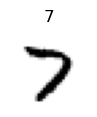``````assert ax.title.get_text() in ('3','7')
test_fig_exists(ax)``````

source

### ToTensor

`` ToTensor (enc=None, dec=None, split_idx=None, order=None)``

Convert item to appropriate tensor class

source

### IntToFloatTensor

`` IntToFloatTensor (div=255.0, div_mask=1)``

Transform image to float tensor, optionally dividing by 255 (e.g. for images).

``````t = (TensorImage(tensor(1)),tensor(2).long(),TensorMask(tensor(3)))
tfm = IntToFloatTensor()
ft = tfm(t)
test_eq(ft, [1./255, 2, 3])
test_eq(type(ft), TensorImage)
test_eq(ft.type(),'torch.FloatTensor')
test_eq(ft.type(),'torch.LongTensor')
test_eq(ft.type(),'torch.LongTensor')``````

source

`` broadcast_vec (dim, ndim, *t, cuda=True)``

Make a vector broadcastable over `dim` (out of `ndim` total) by prepending and appending unit axes

source

### Normalize

`` Normalize (mean=None, std=None, axes=(0, 2, 3))``

Normalize/denorm batch of `TensorImage`

``````mean,std = [0.5]*3,[0.5]*3
mean,std = broadcast_vec(1, 4, mean, std)
batch_tfms = [IntToFloatTensor(), Normalize.from_stats(mean,std)]
tdl = TfmdDL(train_ds, after_batch=batch_tfms, bs=4, device=default_device())``````
``````x,y  = tdl.one_batch()
xd,yd = tdl.decode((x,y))

test_eq(x.type(), 'torch.cuda.FloatTensor' if default_device().type=='cuda' else 'torch.FloatTensor')
test_eq(xd.type(), 'torch.LongTensor')
test_eq(type(x), TensorImage)
test_eq(type(y), TensorCategory)
assert x.mean()<0.0
assert x.std()>0.5
assert 0<xd.float().mean()/255.<1
assert 0<xd.float().std()/255.<0.5``````
``````#Just for visuals
from fastai.vision.core import *``````
``tdl.show_batch((x,y))``